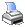﻿ MCL Reference > Miscellaneous Functions > Same( )
 Navigation:  MCL Reference > Miscellaneous Functions > Same( )The function will return a condition expression. The left hand side of the condition expression is an MCL expression. The right hand side of the condition expression is the value of the MCL expression in current start. The operator of the condition expression is default to "=". The MCL expression must be a simple expression without any "And" or "Or" (can contain + - X / ).

Syntax

Same(cExpression,cOperator)

Example

Same('HrDistance( )')

If the above function is applied to a 1650m start, the function will return

'HrDistance( )=1650'

HrAverage('HrSpeed( )',6,'For '+Same('HrDistance( )'))

If the above function is applied to a 1650m start, the function will return

HrAverage('HrSpeed( )',6,'For HrDistance( )=1650'))

HrAverage('HrSpeed( )',6,'For '+Samd('HrDistance( )')+' and '+Same('HrCourse( )'))

If the above function is applied to a 1650m start, the function will return

HrAverage('HrSpeed( )',6,'For HrDistance( )=1650 and HrCourse( )="HV"')